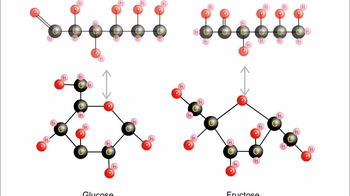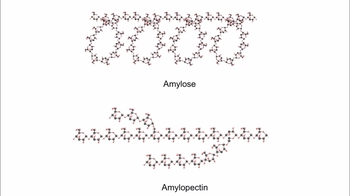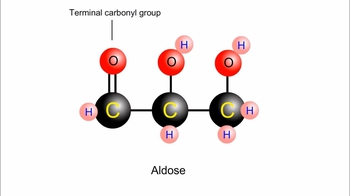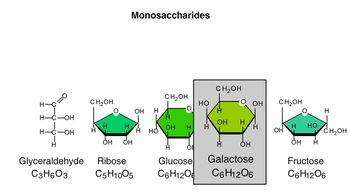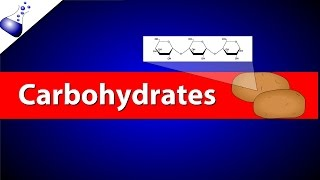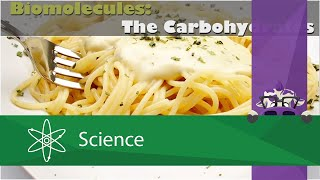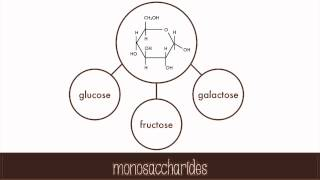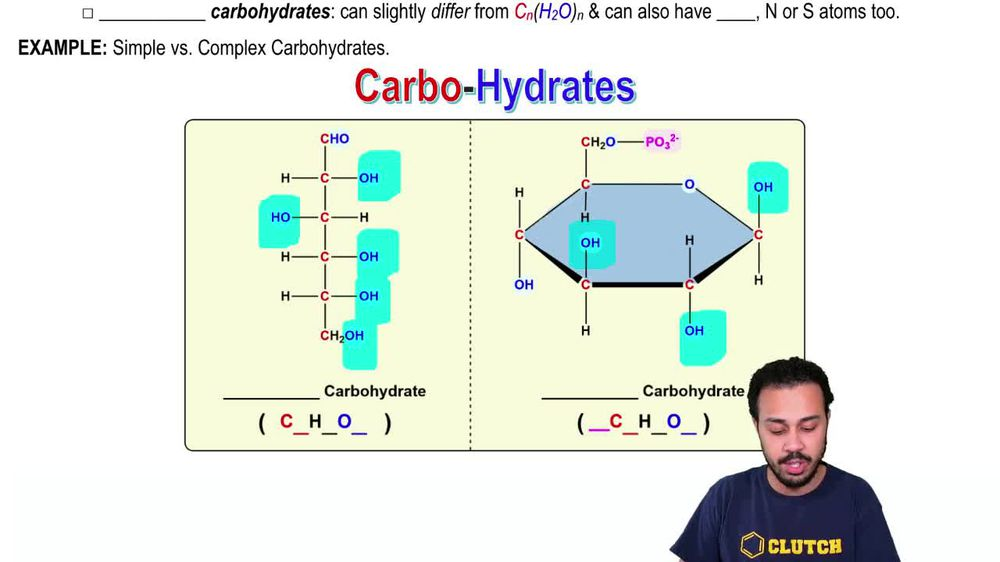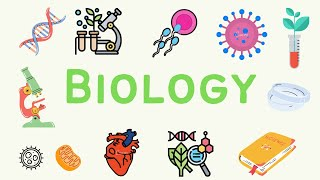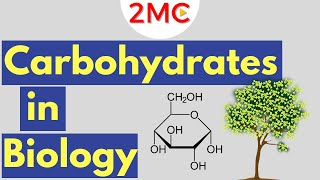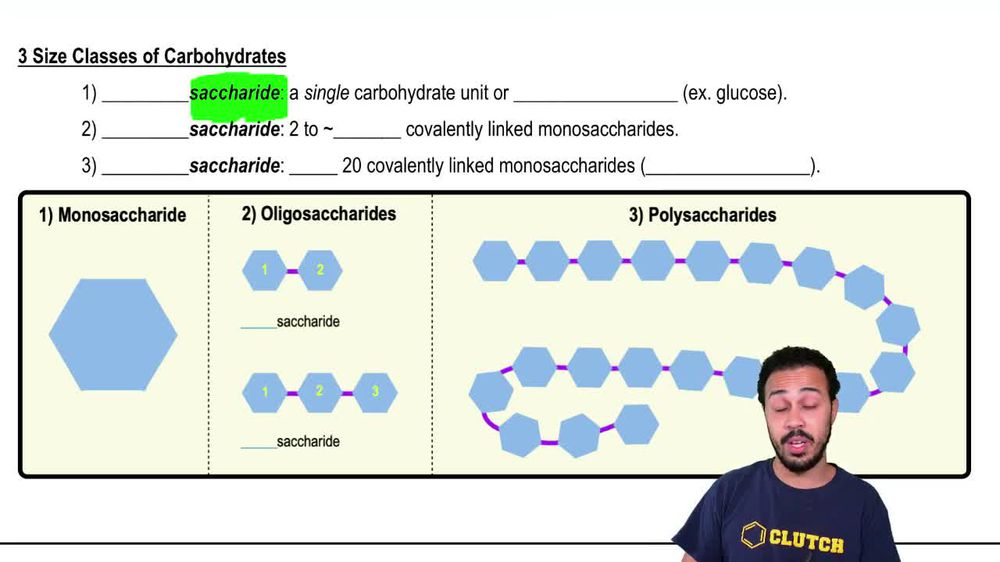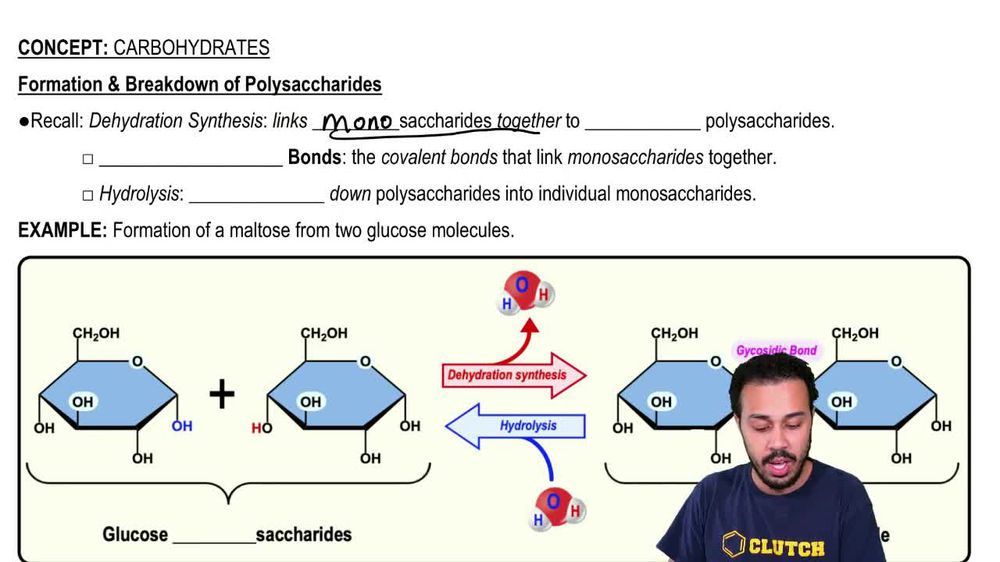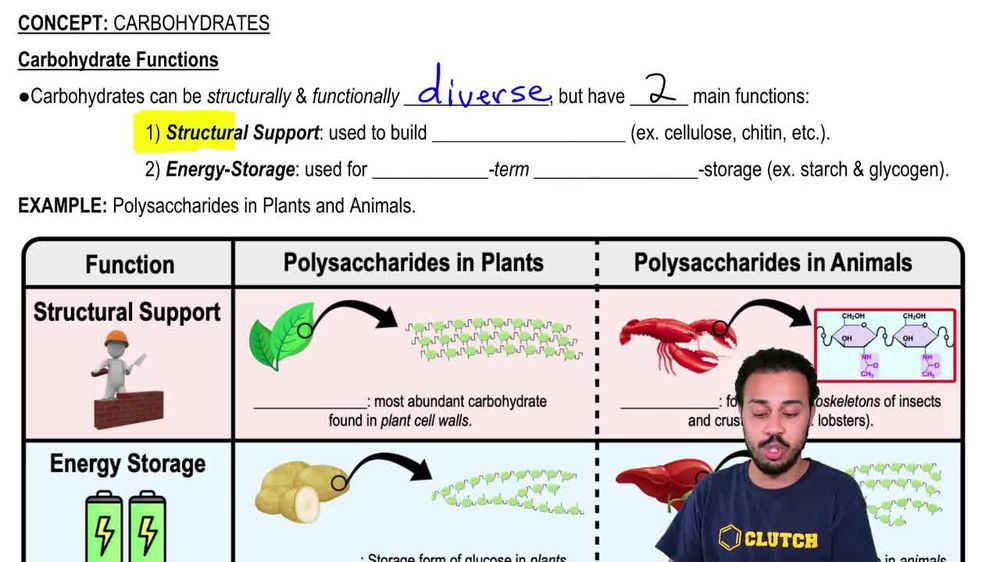Start typing, then use the up and down arrows to select an option from the list.
1. 4. Biomolecules2. Carbohydrates# Carbohydrates

by Jason Amores Sumpter
463 views
1
1
in this video, we're going to begin our lesson on carbo hydrates. Now. Carbohydrates can be defined as carbon based molecules that air hydrated with many hydroxy local groups, which recall are just a functional group that looked like this with an oxygen and hydrogen atom. And so when we take a look at our image down below of carbohydrates, one thing that you'll notice is that there are plenty of these hydroxyl groups throughout their structures, and so that is definitely a characteristic feature of carbohydrates. Now. Carbohydrates are also referred to as sacha rides, and so sacha rides is really the Greek word. That means sugars, and so sugars are carbohydrates. Now, when the term carbo hydrates was originally coined way back in the hundreds, it was actually referring to compounds that had the exact chemical formula of C. N. H. 20 N, where you had some number of carbon atoms being hydrated by some number of water molecules. And that's exactly where it got its name, from the carbo for the car buns and the hydro or the hydrates for the water molecules that air hydrating the carbon atoms. Now it turns out that simple carbohydrates are carbohydrates that fit this chemical formula exactly. For example, glucose is a carbohydrate that fits this chemical formula exactly, and glucose is three most abundant carbohydrate, and it's the one that you guys should all be familiar with, and we'll be able to see an example of glucose down below in our image. But not all carbohydrates fit this chemical formula. Exactly. And so there are some complex carbohydrates and complex carbohydrates are going to be carbohydrates that can slightly differ from this chemical formula here. And they can also have other types of atoms, such as phosphorus, nitrogen or sulfur atoms, to. So let's take a look at our example down below to distinguish between the simple and complex carbohydrates. So notice on the left hand side over here when we take a look at its chemical formula, and we count up the total number of carbon atoms, the total number of hydrogen atoms throughout and the total number of oxygen atoms throughout. What we'll see is that there are a total of six carbon atoms, a total of 12 hydrogen atoms and a total of six oxygen atoms. And so what you'll notice is that there are six water molecules that we can make out of the H 12 06 on those water molecules air hydrating, the six carbon atoms. And so this is going to be a molecule that fits the chemical formula up above. Exactly. And so this is going to be an example of a simple carbohydrate. More specifically, this is the chemical structure of glucose. And once again, glucose is the most abundant carbohydrate. And you should start to recognize its chemical formula of C six H 12 06 Because at some point in your course, you will need to know this chemical formula. Now, over here on the right hand side, what we're showing you is a complex carbohydrate, and we can tell that it's a complex carbohydrate because its chemical formula does not match the one that we have up above and so notice that it has a total of six carbon atoms. But when you count the hydrogen atoms, there are 11 of them. And when you count the oxygen atoms, they're actually nine of them. And there's also one phosphorus atom as well, which you can see up above right here. And so this is a complex carbohydrate, not because it has a circular shape, but because its chemical formula does not fit the one up above Exactly. And so moving forward were mainly gonna be focusing on simple carbohydrates. But it's good for you to also know that complex carbohydrates also do exist. And so this here concludes our introduction to carbohydrates. And we'll get to talk Mawr and Maura about carbohydrates as we move forward in our lessons. So I'll see you all in our next video.